### 激活函数¶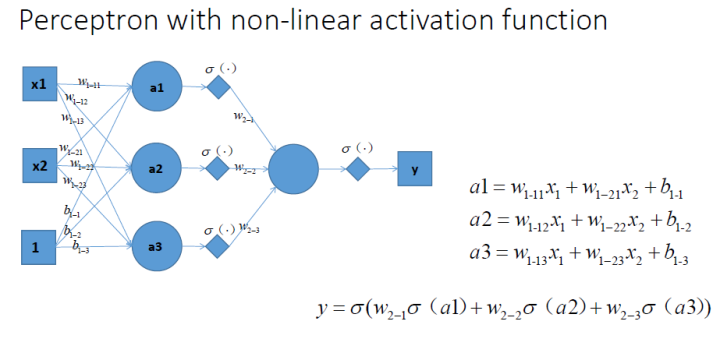### 激活函数的性质¶

• 非线性（应该也可以是线性的，但没有意义）
• 可微（通俗点就是可以求导，梯度下降时需要）

$$z=\sum_{i}w_i x_i+b$$

$$\frac{\partial{L}}{\partial{w_i}} = \frac{\partial{L}}{\partial{\sigma}}\frac{\partial{\sigma}}{\partial{z}} x_i$$

### 常用激活函数及其实现¶

In :
import numpy as np
import matplotlib.pyplot as plt
X = np.linspace(-10, 10, 10000)


#### Sigmoid¶

$$\sigma ( x ) = \frac { 1 } { 1 + e ^ { - x } }$$
In :
Y = 1/(1+np.exp(-X))
plt.plot(X, Y)
plt.grid()
plt.show()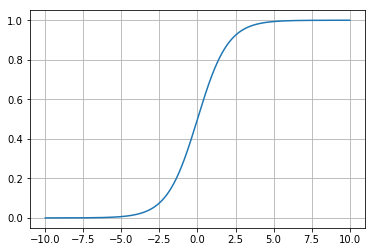Sigmoid 有一个很大的特性，对 Sigmoid 求导会发现，它的导数恰好是 $\sigma'(x)=\sigma(x)(1-\sigma(x))$

cs231n 问及：Consider what happens when the input to a neuron is always positive...What can we say about the gradients on w?

Sigmoid 面临的问题是:

• 饱和时梯度消失，当输入过大或过小，输出都接近于 1，这时导致导数接近于 0，因此出现梯度消失。所以 Sigmoid 对零附近的输入最敏感。
• 非零为中心。$sigmoid(x)$ 的值域为 $(-1, 1)$，会发现 Sigmoid 的导数一定大于 0，通过上面的梯度计算公式，如果一直 $x_i>0$ 或 $x_i<0$，会出现 $w$ 一直为正或者负。
• 指数计算复杂。

#### tanh¶

$$\tanh ( x )=\frac { e ^ { x } - e ^ { - x } } { e ^ { x } + e ^ { - x } }$$
In :
Y = np.tanh(X)
plt.plot(X, Y)
plt.grid()
plt.show()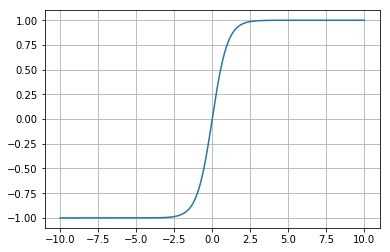$$\sigma' ( x )= \frac { 4 } { \left( e ^ { x } + e ^ { - x } \right) ^ { 2 } }$$

tanh 虽然满足 zero centered，关于原点对称，但是饱和时，仍然会出现梯度消失（梯度接近于 0），而且指数计算复杂。

#### ReLU(Rectified Linear Unit)¶

$$\max ( 0 , x )$$
In :
Y = np.maximum(0, X)
plt.plot(X, Y)
plt.grid()
plt.show()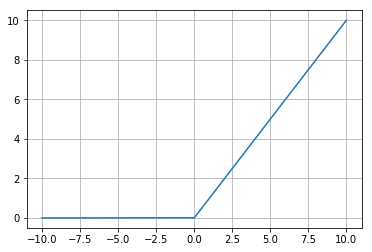ReLU 常用于深度学习中，好处显而易见:

• $x>0$ 不会饱和。
• 计算高效。
• 实际应用的时候收敛速度比 sigmoid/tanh 快很多。
• 比 sigmoid 更像生物上的神经元。

• 非 zero-centered。
• 当 $x<0$，通过上面的梯度公式，梯度直接为 0，也就是梯度消失。即 ReLU 不会被激活，不会更新参数。

#### Leaky ReLU¶

$$f ( x ) = \max ( 0.01 x , x )$$
In :
Y = np.maximum(0.01*X, X)
plt.plot(X, Y)
plt.grid()
plt.show()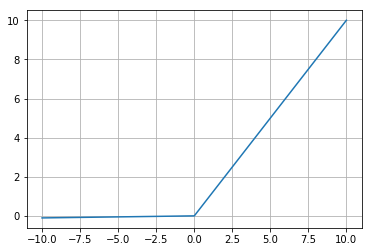ReLU 加上一点正的偏置就是 Leaky ReLU 了，通常用在 GAN 中。优势和面临的问题和 ReLU 一样，但重要的是 Leaky ReLU 解决了 ReLU 的梯度消失问题，当 $x<0$，梯度不会消失。

#### Parametric Rectifier (PReLU)¶

$$f ( x ) = \max ( \alpha x , x )$$

PReLU 将 Leaky ReLU 的偏置设为超参，$\alpha$ 需要优化，当 $\alpha=0.01$ 时就是 Leaky ReLU。反向传播的时候会出现 $\alpha$ 更新权重。

#### Exponential Linear Units (ELU)¶

$$f ( x ) = \left\{ \begin{array} { l l } { x } & { \text { if } x > 0 } \\ { \alpha ( \exp ( x ) - 1 ) } & { \text { if } x \leq 0 } \end{array} \right.$$
In :
alpha = 1
import copy
Y = copy.deepcopy(X)
Y[Y<=0] = alpha*(np.exp(X[X<=0])-1)
plt.plot(X, Y)
plt.grid()
plt.show()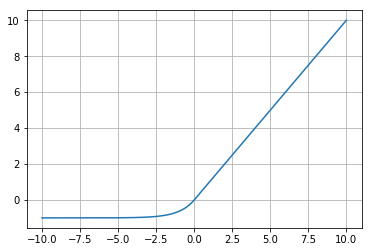• ReLU 的所有优势。
• 改进之处是近似于 zero mean。
• $x$ 非常小时，出现饱和。
• 指数计算复杂。

#### Maxout¶

$$\max \left( w _ { 1 } ^ { T } x + b _ { 1 } , w _ { 2 } ^ { T } x + b _ { 2 } \right)$$

### 激活函数使用技巧¶

• 使用 ReLU 时注意学习率，不能和 Xavier 初始化一起使用。
• 都试一下这几个激活函数: Leaky ReLU / Maxout / ELU。
• 可以尝试 tanh 但不要期望可以得到很好的效果。
• 不要使用 sigmoid，cs231n 重点强调，可能是因为缺点太多。